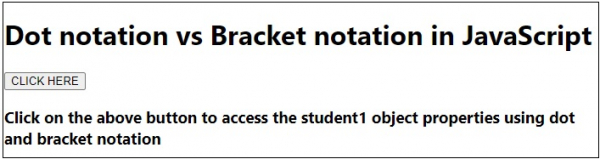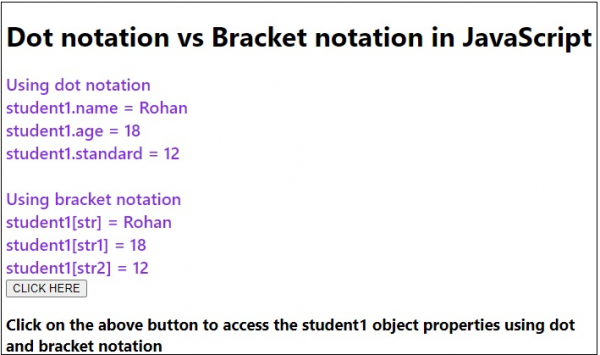# Dot notation vs Bracket notation in JavaScript

JavascriptWeb DevelopmentObject Oriented Programming

The dot notation and bracket notation both are used to access the object properties in JavaScript. The dot notation is used mostly as it is easier to read and comprehend and also less verbose. The main difference between dot notation and bracket notation is that the bracket notation allows us to access object properties using variable.

Following is the code for bracket notation vs dot notation in JavaScript −

## Example

Live Demo

<!DOCTYPE html>
<html lang="en">
<meta charset="UTF-8" />
<meta name="viewport" content="width=device-width, initial-scale=1.0" />
<title>Document</title>
<style>
body {
font-family: "Segoe UI", Tahoma, Geneva, Verdana, sans-serif;
}
.result {
font-size: 20px;
font-weight: 500;
color: blueviolet;
}
</style>
<body>
<h1>Dot notation vs Bracket notation in JavaScript</h1>
<div class="result"></div>
<h3>Click on the above button to access the student1 object properties using dot and bracket notation</h3>
<script>
let resEle = document.querySelector(".result");
let BtnEle = document.querySelector(".Btn");
function Student(name, age, standard) {
this.name = name;
this.age = age;
this.standard = standard;
}
let student1 = new Student("Rohan", 18, 12);
let str = "name",
str1 = "age",
str2 = "standard";
resEle.innerHTML ="Using dot notation <br>student1.name = " + student1.name +       "<br>";
resEle.innerHTML += "student1.age = " + student1.age + "<br>";
resEle.innerHTML +=
"student1.standard = " + student1.standard + "<br><br>";
resEle.innerHTML +=" Using bracket notation <br>student1[str] = " +
student1[str] +"<br>";
resEle.innerHTML += "student1[str1] = " + student1[str1] + "<br>";
resEle.innerHTML += "student1[str2] = " + student1[str2] + "<br>";
});
</script>
</body>
</html>

## Output